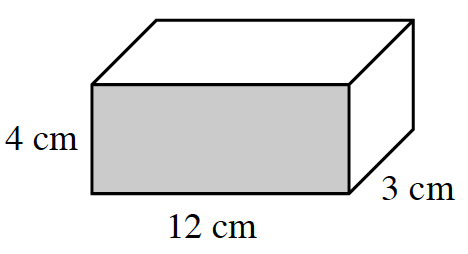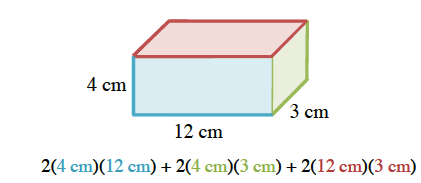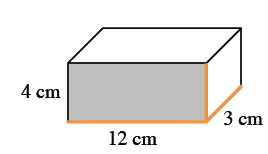### Home > CC2MN > Chapter 9 Unit 9 > Lesson CC2: 9.2.2 > Problem9-66

9-66.Use the rectangular prism shown at right to complete parts (a) through (d) below.  Homework Help ✎

1. Calculate the surface area of the prism. Show all of your subproblems.

To find the surface area, find the area of each face of the prism.

There are $6$ faces in a rectangular prism.
Each of the three different faces shown in the image have an opposite face, so find the area of each of those $3$ faces and multiply it by $2$.2. Explain why the exponent for area units is $2$ (that is, cm2).

How many terms do you multiply together to find area?

To find area, you multiply two dimensions.
Just as $(x)(x)=x^2,(\text{cm})(\text{cm})=\text{cm}^2$.

3. Calculate the volume of the prism.

To find the volume of a rectangular prism, multiply the base, height, and width together.

$\text{Volume}=(12\ \text{cm})(4\ \text{cm})(3\ \text{cm})$4. Explain why the exponent for volume units is a $3$ (that is, $\text{cm}^{3}$).

See part (b).